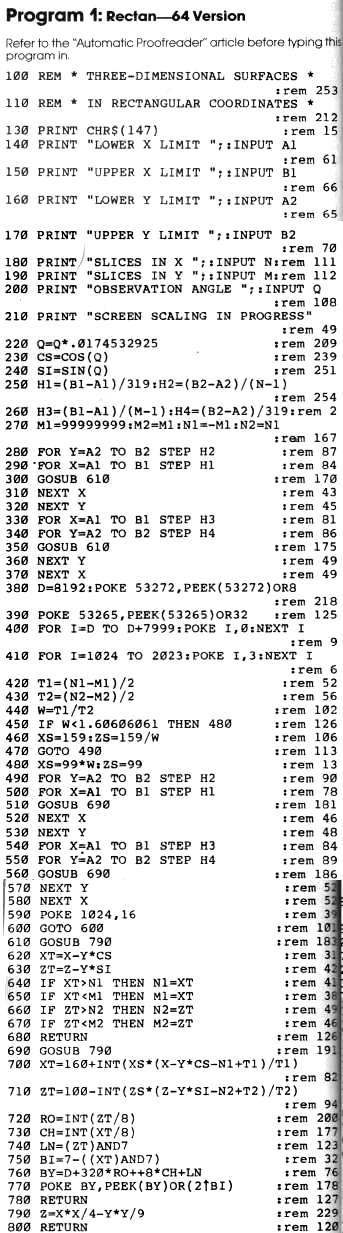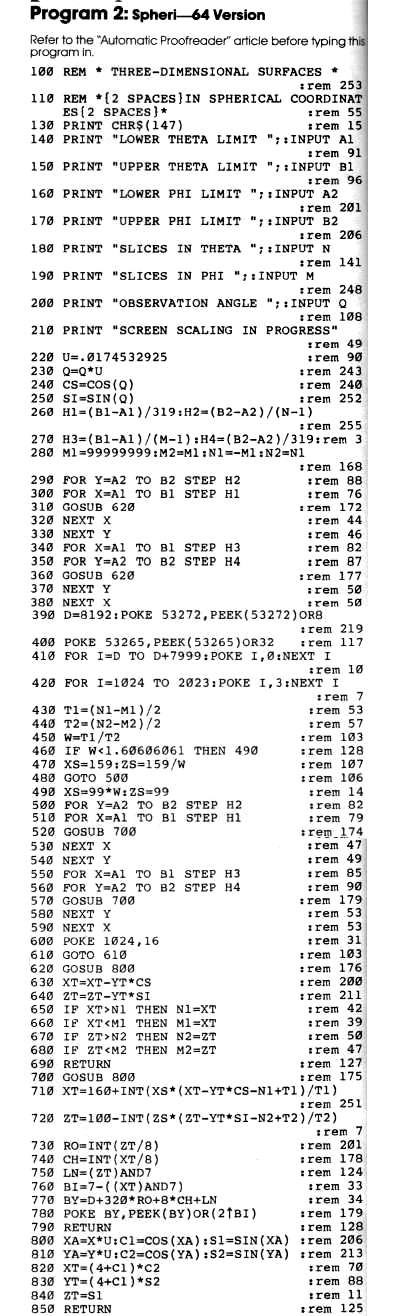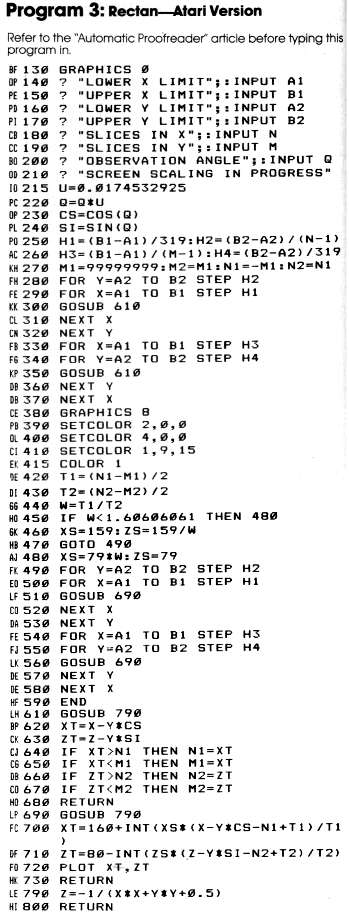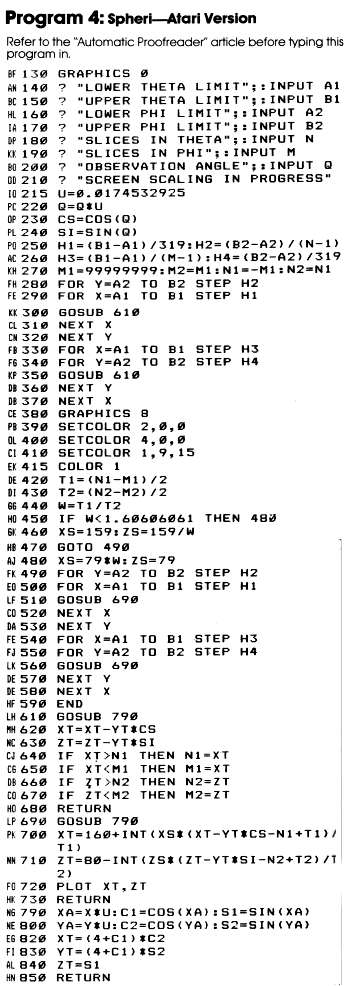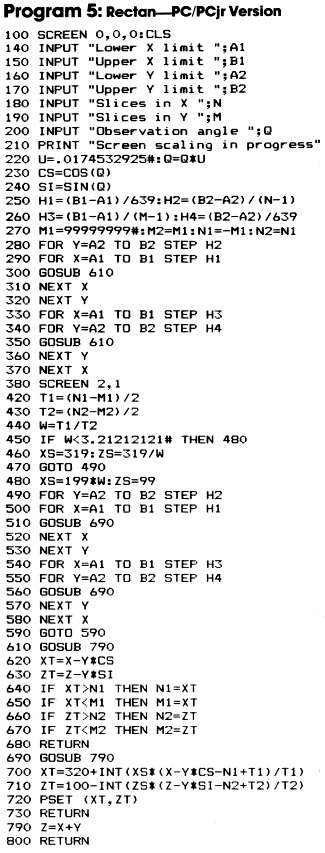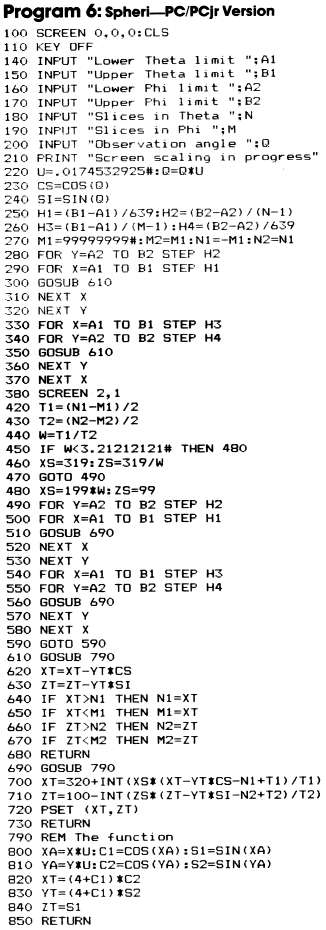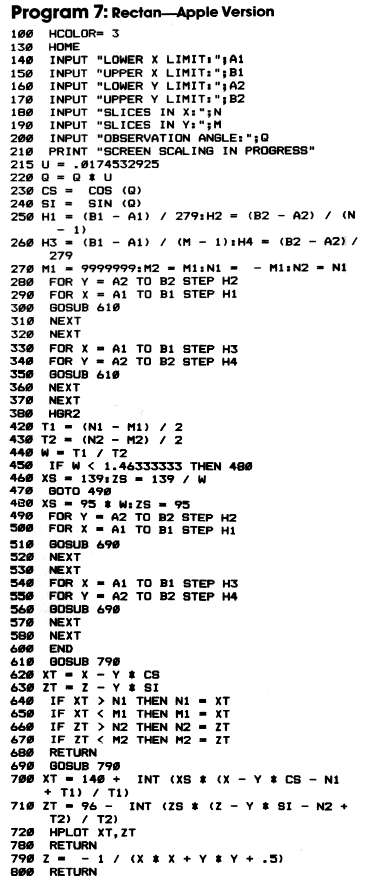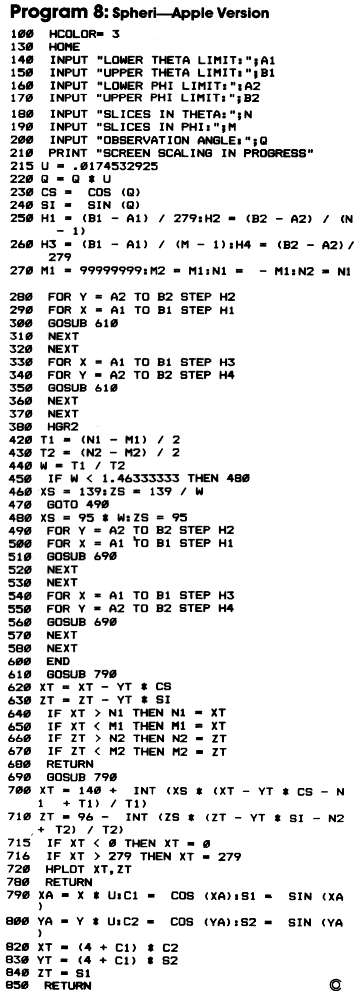`COMPUTE! ISSUE 48 / MAY 1984 / PAGE 58`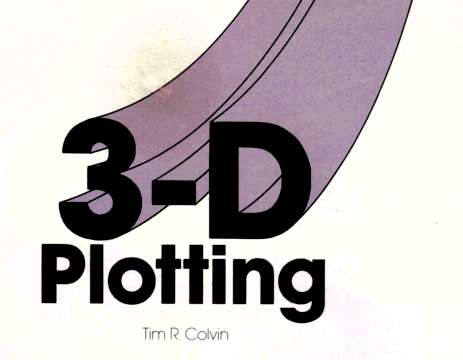# 3-D Plotting

Tim R. Colvin

How many times have you seen beautiful three-dimensional graphics in the ads for video monitors and printers? Now, with these easy-to-use programs, you can create three-dimensional images of your own. Versions are included for the Commodore 64, Atari, Apple, IBM PC and PCjr.

These two programs, "Rectan" and "Spheri," will plot three-dimensional figures using information which you provide.

You don't really need to delve into the mathematics which produce the images. You can just fiddle with the examples given to produce many effective displays. Let's look at some graphic examples. First type in each program and SAVE it to tape or disk.

Then LOAD Rectan. To have Rectan draw a hyperbolic paraboloid, or "saddle function" (it resembles a riding saddle), replace line 790 with:

790 Z-X*X/4-Y*Y/9

and give the following inputs:

-2,2,-3,3,25,25,45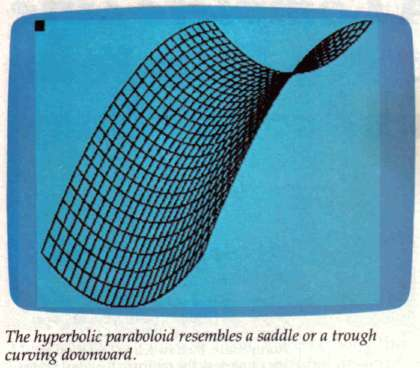For another interesting design, use:

790 Z=-l/(X*X+Y*Y+.5)

and give the following inputs:

-1,1,-1,1,20,20,45

The program will print SCREEN SCALING IN PROGRESS. The program is scaling the image to fit on the screen, which can require a lot of time. The rule is: The more complicated the description of the surface, the longer this step takes.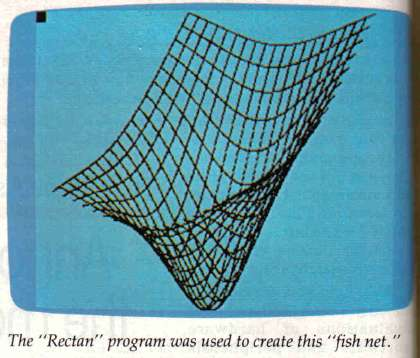## The Plotting Begins

When the previous step is completed, the screen will clear and turn cyan. The high-resolution plotting now begins. When the plot is finished, the color of the top left corner of the screen will change color. The program is locked in a loop so you can look at your creation. When you have finished looking at the display hold down RUN/ STOP and hit RESTORE.

## A Spheri Demonstration

To see a torus (doughnut shape), type NEW to clear memory. Then LOAD Spheri, replace lines 820-840 with:

```820 XT=*(4+C1)*C2
830 YT=(4+C1)*S2
840 ZT=S1
```

and give the following inputs:

0,360,0,360,25,25,45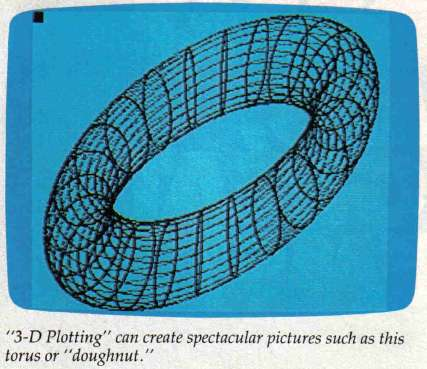For a sphere, use:

```820 XT=C1*C2
830 YT=C1*S2
840 ZT=S1
```

and give the following inputs:

0,360,0,180,15,15,45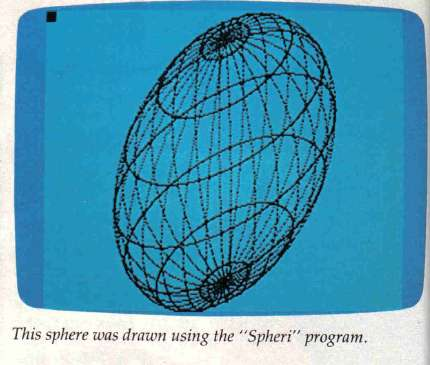## An Illusion Of Depth

These programs use rectangular and spherical coordinate systems to create an illusion of depth in the screen image. You're probably familiar with the X-Y coordinate system used to specify the location of a point on a flat surface. For example, in Figure 1 the point is located five units over on the X axis and six units up on the Y axis. The point is said to be at location 5,6.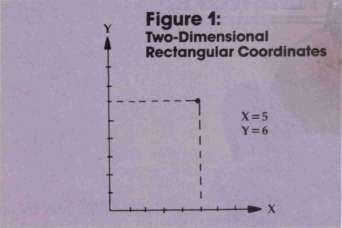This simple system works well for specifying the location of a point in a two-dimensional design on a flat surface, but for 3-D plotting you need a third coordinate.

Several coordinate systems are commonly used to plot three-dimensional surfaces. The particular coordinate system you should use depends on the shape you want to draw. Any system can be used, but if you choose the right system, you can simplify your calculations considerably.

## A Simple Solution

The easiest system to understand is just an extension of the rectangular (X-Y) coordinates you are already familiar with. All you need to add is a third coordinate (Z) for the third dimension. For example, the point in Figure 2, below, is located five units out on the X axis, six units over on the Y axis, and four units up on the Z axis. The point is said to be at location 5,6,4.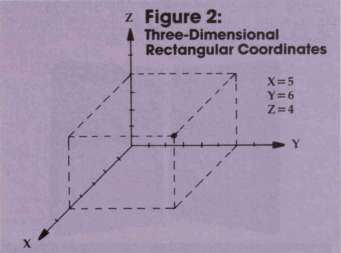## A System For The Stars

On the other hand, if the design you wish to draw is roughly the shape of a sphere, you should use spherical coordinates. In that system, a point is described by two angles and a distance from the origin. For example, astronomers use spherical coordinates to describe the position of a star relative to the earth. The azimuthal angle of the star, designated by the Greek letter "theta", is the direction you must face to view the star. If north is taken to be zero degrees, then a star that lies due east has an azimuthal angle of 90 degrees. The elevation angle, designated by the Greek lette "phi", specifies how much you must tilt your head back to look directly at the star. If the horizon is taken to be zero degrees, a star that is directly overhead has an elevation angle of 90 degrees. Finally, the radial distance, designated by the letter r, is the distance between the earth and the star.

Using spherical coordinates, the point shown in Figure 2 has an azimuthal angle of 50.2 degrees, an elevation angle of 33.7 degrees, and a radial distance of 8.77 units, as shown in Figure 3.

Despite the fine graphics they produce, these programs have a couple of limitations. Screen pixels are taller than they are wide, which makes spheres look slightly less round than they should Also, we see the surface as if it were transparent and contour lines were drawn on it. A more advanced program (such as those available commercially) would remove lines that we couldn't see if the surface were not transparent.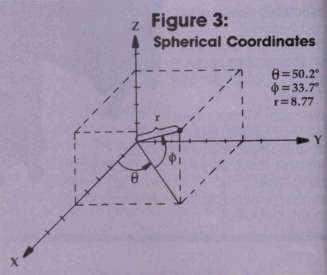## The Mathematics Of 3-D Plotting

"Rectan" plots surfaces using rectangular coordinates (x,y,z). The values for x and y are specified; the value of z is then given by z = f(x,y) for some function f.

To use Rectan, specify the function f(x,y) in line 790. For example, z = x*x/4-y*y/9 defines a hyperbolic paraboloid.

"Spheri" plots surfaces using spherical coordinates. This method describes a point on the surface using three parameters: radial distance from the origin, r; azimuthal angle, theta; and elevation angle, phi.

To use Spheri, specify x,y, and z (called XT,YT, and ZT in lines 820-840) as functions of r, theta, and phi in lines 820-840.

## Parameters And Slices

Both programs are structured the same. You specify parameter ranges. In Rectan these are for x and y; in Spheri, for [theta] and [phi].

Next enter the number of slices for the parameters. Each slice corresponds to a contour line on the surface. A contour line is where one of the parameters is held constant.

Finally, you specify an observation angle. This is the angle which allows you to see a three-dimensional surface on a two-dimensional video screen. The most commonly used angle is 45 degrees.

If you'd like any technical information, or if you have a particular surface in mind but don't know how to write an equation for it, please write to:

Tim R. Colvin 1414 San Remo Dr. Pacific Palisades, CA 90272